# GSEB Solutions Class 11 Physics Chapter 8 Gravitation

Gujarat Board GSEB Textbook Solutions Class 11 Physics Chapter 8 Gravitation Textbook Questions and Answers.

## Gujarat Board Textbook Solutions Class 11 Physics Chapter 8 Gravitation

### GSEB Class 11 Physics Gravitation Text Book Questions and Answers

Question 1.
(a) You can shield a change from electrical forces by putting it inside a hollow conductor. Can you shield a body from gravitational influence of neacby matter by putting it inside a hollow sphere or by some other means?
(b) An astronaut inside a small space ship orbiting around the Earth cannot detect gravity. If the space station orbiting around the Earth has a large size can he hope to detect gravity?
(c) If you compare the gravitational force on the Earth due to the Sun to that due to the moon, you would find that the Sun’s pull is greater than moon’s pull. (You can check this yourself using the data available in the succeeding exercises.) However, the tidal effect of the moon’s pull is greater than the tidal effect of the Sun, why?
(a) No. Electrical forces depend upon the nature of the intervening medium while the gravitational forces don’t depend upon the nature of the intervening medium, hence such shielding acts are not possible in case of gravitation i.e. gravity screens are not possible.

(b) Yes, he can hope to detect gravity if the size of the spaceship is extremely large, then the magnitude of the gravity will become appreciable and hence the gravitational effect of the spaceship may become measurable.

(c) Earth-moon distance is very smafl as compared to Earth-Sun distance. Tidal effect is ¡nvcrsy proportional to the cube of the distance i.e. it is not governed by inverse square law like the gravitational force (which obeys inverse square law). Therefore, tidal effect of moon is larger than that due to the sun.Question 2.
Choose the correct alternatives:
(a) Acceleration due to gravity increases/decreases with increasing altitude.
(b) Accelaration due to gravity increases/decreases with increasing depth (assume the Earth to be a sphere of uniform density).
(c) Accelaration due to gravity is independent of the mass of the Earth/mass of the body.
(d) The formula – GMm ($$\frac{1}{r_{2}}-\frac{1}{r_{1}}$$) is more less accurate than the formula mg (r2 – r1) for the difference of potential energy between two points r1 and r2 distance away from the centre of earth.
(a) Acceleration due to gravity decreases with increasing altitude.

(b) Acceleration due to gravity decreases with increasing depth.

(c) Acceleration due to gravity is independent of the mass of the body.

(d) The formula -GMm ($$\frac{1}{\mathrm{r}_{2}}-\frac{1}{\mathrm{r}_{1}}$$) is more accurate than the formula mg (r2 – r1) for the difference of potential energy between two points r1 and r2 distance away from the centre of earth.

Question 3.
Suppose there existed a planet that went around the Sun twice as fast as the Earth. What would be its orbital size as compared to that of the Earth?
Let r = radius of the orbit of Earth around Sun.
Me = Mass of Earth
Ms = Mass of Sun
ω = angular speed of the Earth around the Sun
ω’ = angular speed of the planet around the Sun
r’ = radius of the orbit (orbital size) of the planet around the Sun.
The centripetal force required for revolution of Earth around Sun is provided by gravitational force between Sun and Earth.i.e. orbital size of planet = 0.63 times the orbital size of Earth.
i.e. planet’s orbital size is smaller than the orbital size of Earth by a factor of 0.63.Question 4.
If one of the satellites of Jupiter has an orbital period of 1.769 days and the radius of the orbit is 4.22 × 108 m. Show the mass of Jupiter is about one thousandth that of Sun?
Let MJ = Mass of Jupiter = ?
MS = Mass of Sun = 2 × 1030 kg
T = Time period of I0 satellites of Jupiter = 1.769 days
= 1.769 × 24 × 3600s
= 15.2841 × 104s
r = radius of its orbit around Jupiter = 4.22 × 108 m.
G = 6.67 × 10-11 Nm2kg-2
To prove MJ = $$\frac{1}{1000}$$ Ms
Using the relation,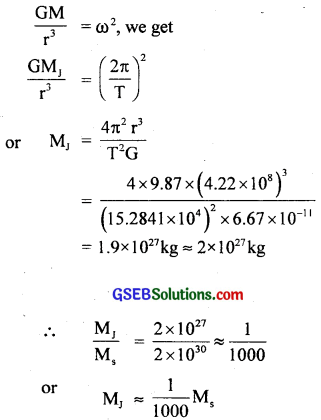i.e. Mass of Jupiter is about one thousandth of the mass of sun. Hence proved.

Question 5.
Let us assume that our galaxy consists of 2.5 × 1011 stars each of one solar mass. How long will a star at a distance of 50,000 light years from the galactic centre take to complete one revolution? Take the diameter of the milky way to be 10s light years?
One solar mass = 2 × 1030 kg.,
1 light year = 9.46 × 1015 m
Let M = Mass of stars in the galaxy
= 2.5 × 1011 × 2 × 1030 kg
= 5 × 1041 kg
r = radius of orbit of a star.
= Distance of a star from galactic centre
= 50,000 light years.
= 50,000 × 9.46 × 1015m.
G = 6.67 × 10-11NM-2kg-2
T = Time of one revolution = ?
Diameter of Milky Way = 105 light years
Using the relation,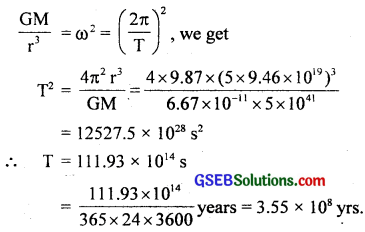Question 6.
Choose the correct alternative:
(a) If the zero of potential energy is at infinity, the total energy of an orbiting satellite is negative of the kinetic/ potential energy.
(b) The energy required to rocket an orbiting satellite out of Earth’s gravitational influence is more/less than the energy required to project a stationary object at the same height (as the satellite) out of Earth’s influence.
(a) Kinetic energy
(b) less.

Question 7.
Does the escape velocity of a body from the Earth depend on (a) the mass of the body, (b) the location from which it is projected, (c) the direction of projection, (d) the height of the location from where 4he body is launched? Explain your answer?
(a) No. We know that the escape velocity of the body is given by Ve = $$\frac{\sqrt{2GM}}{R}$$, where M and R are mass and radius of Earth. Thus clearly, it does not depend on the mass of the body as Vc is independent of it.

(b) Yes. We know that Ve depends upon the gravitational potential at the point from where the body is launched. Since the gravitational potential depends on the latitude and height of the point, therefore the escape velocity depends on the location of the point from where it is projected. It can also be experienced as:
Ve = $$\sqrt{2gr}$$. As g has different values at different heights. Therefore Ve depends upon the height of location.

(c) No, it does not depend on the direction of projection as Ve is independent of the direction of projection.

(d) Yes, it depends on the height of location from where the body is launched as explained in (b).

Question 8.
A comet orbits the sun in highly elliptical orbit. Does the comet has a constant (a) linear speed, (b) angular speed, (c) angular momentum, (d) kinetic energy, (e) potential energy and (f) total energy throughout its orbit? Neglect any mass loss of the comet when it comes very close to sun?
All quantities vary over an orbit except angular momentum, and the total energy which can be explained as follows:
(a) According to the law of conservation of angular mementun, the planet (comet) moves faster when it is close to the sun and moves slower when it is farther away from the sun. Hence linear speed of the comet does not remain constant.

(b) Angular speed of the comet also varies slightly.

(c) According to the law of conservation of angular momentum, the angular momentum of the comet remains constant.

(d) Since the linear speed of the comet around the Sun changes continuously, so its kinetic energy also changes continuously.

(e) Potential energy depends upon the distance between the Sun and the comet, hence P.E. changes in the elliptical orbit as the distance between Sun and the comet changes continuously.

(f) As total energy is the sum of K.E. and P.E., so the total energy always remains constant according to the law of conservation of energy.Question 9.
Which the following symptoms is likely to afflict an astronaut in space, (a) swollen feet, (b) swollen face, (c) headache and (d) orientational problem?
(b), (c), (d) i.e. swollen face, headache and orientational problem are the symptoms likely to afflict an astronaut in space.

Question 10.
The gravitational intensity at the centre of a hemispherical shell of uniform mass density has the direction indicated by the arrow (see in the fig.) (i) a, (ii) b, (iii) c, (iv) zero?If we complete the hemisphere to a sphere as shown in fig. then at both the points P and C, potential is constant and hence gravitation intensity (I) at the centre of the spherical shell will be zero as it is a shell now (iii) (c) is correct.

Question 11.
For the above problem, the direction of the gravitational intensity at an arbitrary point P is indicated by the arrow (i) d, (ii) e, (iii) f, (iv) g?
At point P, Potential is constant and hence force = 0. Therefore (f) and (e) are correct.Question 12.
A rocket is fired from the Earth towards the Sun. At what distance from the Earth’s centre is the gravitational force on the rocket zero? Mass of the Sun = 2 × 1030 kg.,Mass of Earth = 6.0 × 1024 kg. Neglect the effect of the other planets etc. (Orbital radius = 1.5 × 1011 m)
Let P be a point at a distance r from earth’s centre where gravitational force due to Sun and Earth is equal and hence gravitational force on the rocket is zero.
Let x = distance between Sun and Earth = radius of orbit of earth
Mass of Sun, Ms = 2 × 1030 kg
Mass of Earth, Me = 6 × 1024 kg
Also let m = mass of the rocket
∴ At P, Gravitational force between sun and rocket = Gravitational force between earth and rocket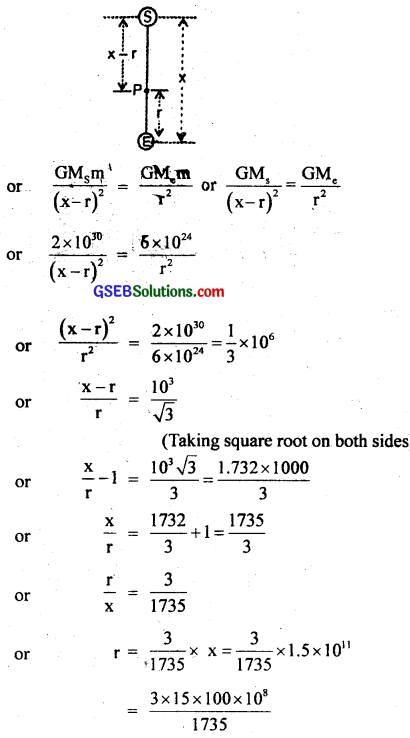or r = 2.594 × 108 m
= 2.6 × 108 m from Earth.

Question 13.
How will you weigh the Sun i.e. estimate its mass? You will need to know the period of one of its planets and the radius of the planetary orbit. The mean orbital radius of the Earth around the Sun is 1.5 × 108 km. Estimate the mass of the Sun?
We know that Earth revolves around the Sun in an orbit of radius 1.5 × 1011 m and completes one revolution around the Sun in 365 days.
∴ R = radius of orbit of Earth = 1.5 × 1011 m
T = Time period of Earth around the Sun
= 365 days
= 365 × 24 × 60 × 60s.
G = 6.67 × 10-11Nm2kg-2
We know thator Ms = 2.0 × 1030 kg
∴ Mass of sun = 2.0 × 1030 kg.Question 14.
A Saturn year is 29.5 times the Earth year. How far is the Saturn from the Sun if the Earth is 1.50 × 108 km away from the Sun?
According to the Kepler’s third law, we know that the square of the time period of revolution of a planet about Sun is α (semi-major axis)3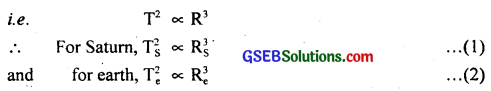Dividing (1) by (2), we get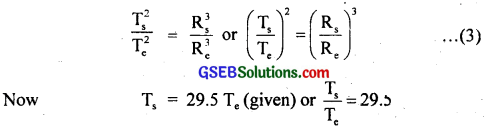and Re = distance of earth from Sun = 1.5 × 108 km
Rs = distance of saturn-from Sun = ? …………………. (4)
∴ From (3) and (4), we get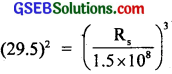or $$\mathrm{R}_{\mathrm{s}}^{3}$$ = (29.5)2 × (1.5 × 108)3
or $$\mathrm{R}_{\mathrm{s}}^{3}$$ = (2.937 × 1027 km3)
∴ Rs = (2.937 × 1027)1/3
= 1.43 × 109 km. = 1.43 × 1012 m.

Question 15.
A body weighs 63 N on the surface of Earth. What is the gravitational force on it due to the Earth at a height equal to half the radius of Earth?
Here, h = height above earth’s surface = $$\frac{R}{2}$$,
where, R = radius of earth.
Now we know that the acceleration due to gravity at a height ‘h’ above earth’s surface (gh) is given byLet m = mass of the body
If w and wh be its weight at Earth’s surface and at a height above Earth’s surface respectively. Then
w = mg = 63 N …………….. (given)
and wh = mgh = m × $$\frac{4}{9}$$g = $$\frac{4}{9}$$ mg
= $$\frac{4}{9}$$ × 63 = 28 N
∴ wh = 28 N.Question 16.
Assuming the Earth to be a sphere of uniform mass density, bow much would body weigh halfway down to the centre of Earth if it weighed 250 N of the surface?
Let g, gd be the acceleration due to gravity on Earth’s surface and at a depth ‘d’ from Earth’s surface respectively.
Also let W and Wd be the weight of a body on Earth’s surface and at depth ‘d’ respectively.
∴ W = mg = 250 N …………………. (i)
and Wd = mgd ………………….. (ii)
Now we know that gd = g(1 – $$\frac{d}{R}$$) ……………….. (iii)
Here, d = $$\frac{R}{2}$$, R = radius of Earth …………………. (iv)
∴ from (iii) and (iv), we get
gd = g(1 – $$\frac{R/2}{R}$$) = g(1 – $$\frac{1}{2}$$)
= g × $$\frac{1}{2}$$ = $$\frac{g}{2}$$ ……………………….. (v)
∴ Wd = mgd = m × $$\frac{g}{2}$$ [by using (v)]
= $$\frac{1}{2}$$ mg = $$\frac{1}{2}$$W = $$\frac{1}{2}$$ × 250 = 125 N.
∴ Weight of the body halfway down to the centre of Earth = 125 N.

Question 17.
A rocket is fired vertically with a speed of 5 km s-1 from the Earth’s surface. How far from the Earth does the rocket go before returning to the Earth?
Mass of the Earth = 6.0 × 1024 kg, mean radius of Earth = 6.4 × 106 m, G = 6.67 × 10-11 Nm2kg-2.
Answrer:
Let y be the initial speed of the rocket which reaches a height h above the surface of Earth where its velocity vanishes i.e. becomes zero.
If m be the mass of the rocket, then its total energy at Earth’s surface is
K.E. + P.E. = $$\frac{1}{2}$$mv2 – $$\frac{GMm}{R}$$ ……………….. (i)
where M = mass of Earth
G = Universal gravitational constant
At highest point, K.E. = 0 (∵ velocity = 0)
and P.E. = – $$\frac{GMm}{R+h}$$ …………………… (ii)
∴ Total energy of the rocket at a height h
= K.E. + P.E.
= 0 + P.E. = P.E. = – $$\frac{GMm}{R+h}$$ ………………….. (iii)
According to the law of conservation of energy,or 2gRh = v2 (R + h)
or Rv2 = 2ghR – v2h = h(2gR – v2)
∴ h = $$\frac{R v^{2}}{2 g R-v^{2}}$$ ……………………… (iv)
Here, v = 5 km s-1 = 5000 ms-1 (given)
R = 6.4 × 106m.
Putting these values in equation (iv), we get= 1.6 × 106m = 1600 km.
∴ Distance from centre of Earth
= R + h = 6.4 × 106 + 1.6 × 106
= 8.0 × 106m.Question 18.
The escape speed of a projectile on Earth’s surface is 11.2 km s-1. A body is projected out with thrice this speed. What is the speed of the body far away from the Earth? Ignore the presence of Sun and other planets?
Note: (In this type of problems, use of law of conservation of energy is the most convenient tool.)
Let v and v be the initial and final speed of the body i.e. its speed of projection and speed at a point far away from Earth.
Also Let m = mass of the body
∴ The initial K.E. of the body = $$\frac{1}{2}$$mv2
∴ The initial P.E. of the body (on Earth’s surface) = – $$\frac{GMm}{R}$$
where, M, R = Mass and radius of Earth
Final P.E. of the body (at ∞) = 0
Final K.E. of the body (at ∞) = $$\frac{1}{2}$$mv2According to the principle of conservation of energy
initial K.E. + initial P.E. = Final K.E. + Final P.E.
or $$\frac{1}{2}$$mv2 – $$\frac{GMm}{R}$$ = $$\frac{1}{2}$$mv2 + 0
or $$\frac{1}{2}$$mv2 = $$\frac{1}{2}$$mv2 – $$\frac{GMm}{R}$$
Also Let, ve = escape velocity
$$\frac{1}{2}$$mv2e = $$\frac{GMm}{R}$$ ……………………… (ii)
∴ from (iii) and (iv), we get
v2 = (3ve)2 – v2e = 9v2e – v2e = 8v2e
= 8 × (11.2)2
or v = $$\sqrt{8 \times(11.2)^{2}}$$ = $$\sqrt{8}$$ × 11.2
= 2 × 1.414 × 11.2
= 31.68 kms-1
∴ speed of the body far away from the Earth,
v = 31.68 kms-1
= 31.7 kms-1.Question 19.
A satellite orbits the Earth at a height of 400 km above the surface. How much energy must be expended to rocket the satellite out of the Earth’s gravitational influence? Mass of the satellite = 200 kg, mass of the Earth = 6.0 × 1024 kg, radius of the Earth = 6.4 × 106 m, G = 6.67 × 10-11 Nm2 kg-2?
Let M, R be the mass and radius of Earth.
Let m = mass of satellite orbiting around Earth at a height h above Earth’s surface.
v = its velocity in the orbit at a height h = orbital velocity.
∴ K.E. of the satellite in the orbit = $$\frac{1}{2}$$mv2
P.E. of the satellite at a height h = – $$\frac{GMm}{R+h}$$
Thus, total energy of the orbiting satellite is given by K.E. + P.E.when it is just removed out of the gravitational pull of Earth (at ∞), its gravitational P.E. will be zero and its K.E. will also be zero.
∴ The final energy of the satellite if it is out of Earth’s gravitational influence = 0.
∴ Energy when it is orbiting at a height h + Energy to be imparted = 0. (applying the law of conservation of energy)
∴ E = Energy to be imparted to put the satellite out of the Earth’s gravitational influence = – energy of the orbiting satellite
= -(-$$\frac{1}{2}$$ $$\frac{GMm}{R+h}$$) = $$\frac{1}{2}$$ $$\frac{GMm}{R+h}$$
here, h = 400 km = 400 × 103 m, R = 6400 × 103 m, G = 6.67 × 10-11 Nm2 kg-2, M = 6 × 1024 kg, m = 200 kg
= 5.885 × 109J = 5.9 × 109 J.Question 20.
Two stars each of 1 solar mass (= 2 × 1030 kg) are approaching each other for a head on collision. When they are at a distance 109 km, their speeds are negligible. What is the speed with which they collide? The radius of each star is 104 km. Assume the stars to remain undistorted unitl they collide. (Use the known value of G)
Here, mass of each star, M = 2 × 1030 kg = one solar mass.
Initial distance between two stars, r = 109 km = 1012 m
Size of each star = radius of each star, R = 104 km = 107 m
Let v = speed with which they just touch (collide) with each other,
u = initial speeds of two stars when they are far away = 0
∴ Potential energy (P.E.) of one star w.r.t. another when they are at a distance r is given byKinetic energy (K.E.) at this distance = 0
∴ Total initial energy = K.E. + P.E. = – – $$\frac{\mathrm{GM}^{2}}{\mathrm{r}}$$
∴ According to the law of conservation of energy,
Total initial energy = Final K.E. + Final P.E.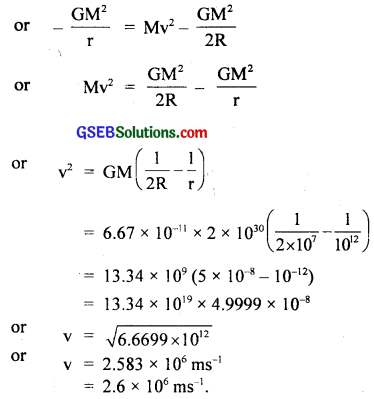Question 21.
Two heavy spheres each of mass 100 kg and radius 0.10 m are placed 1.0 m apart on a horizontal table. What is the gravitational force and potential at the mid-point of the line joining the centres of the spheres? Is an object placed at that point in equilibrium? If so, is the equilibrium stable or unstable?
Let two spheres be placed at points A and B respectively.
r = distance between them = AB = 1m
O = Mid point of the distance AB
= $$\frac{1}{2}$$ × 1m = 0.5 m
∴ AO = OB
= $$\frac{1}{2}$$ × 1m = 0.5 m
M = mass of each sphere = 100 kg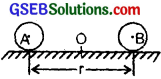We know that the gravitational force between two bodies at a distance d is given by
F = $$\frac{\mathrm{GMm}}{\mathrm{d}^{2}}$$
∴ If FA and FB be the gravitational force at O due to spheres at A and B respectively, then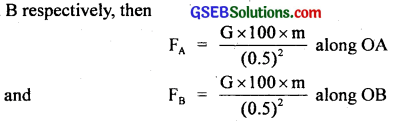Now as |Fa| = |Fb| and they act in opposite direction, so the net force at O = 0 ⇒ zero force at point O means that a body placed at O experiences no force, hence it is in equilibrium, but the equilibrium is unstable as a small displacement in A or B will disturb the equilibrium which is not restored immediately.
Also we know that gravitational potential is given by the formula
= – $$\frac{GM}{d}$$
∴ If VA and VB be the gravitational potentials at point O i.e. mid-point of the line joining the centres of two spheres, then
V = VA + VB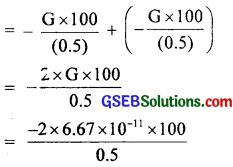= -2.7 × 10-8 J kg-1
= 2.7 × 10-9 J kg-1
Thus, an object placed at the mid-point is in an unstable equilibrium.